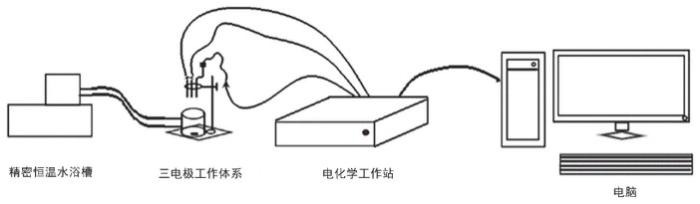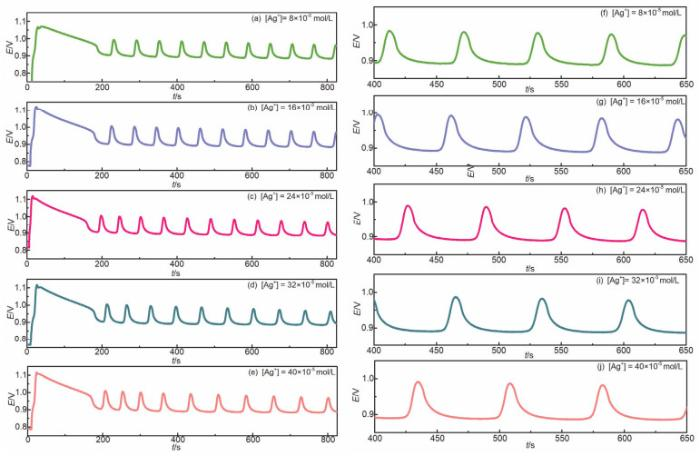## Redesign of Belousov-Zhabotinsky (B-Z) Reaction, an Experiment in Physical Chemistry

Hu Xin, Chen Ze, Yan Tao, Hu Kai, Zhao Faqiong, Huang Chi, Liu Yuwen,

 基金资助: 武汉大学实验教学中心开放实验项目.  WHU-2019-XYKF-07武汉大学2019大学生学科竞赛立项项目.  201921Abstract

The classical kinetic experiment in physical chemistry, Belousov-Zhabotinsky (B-Z) oscillating reaction, was redesigned. The mathematical treatment of nonlinear complex reaction kinetics based on the reaction mechanism and the application of B-Z reaction in the detection of silver ions are introduced in the new experimental scheme. The new scheme can deepen students' understanding of nonlinear non-equilibrium kinetics and dissipative structure. Moreover, the numerical method dealing with the complex kinetics can be used in future scientific research and practice.

Keywords： B-Z reaction ; Oscillation ; Nonlinear kinetics ; FKN mechanism ; Detection of silver ion

Hu Xin. Redesign of Belousov-Zhabotinsky (B-Z) Reaction, an Experiment in Physical Chemistry. University Chemistry[J], 2020, 35(4): 137-144 doi:10.3866/PKU.DXHX201912010

B-Z反应体系是典型的非平衡态非线性热力学体系，体系中作为氧化剂的BrO3在金属离子的催化下，与还原剂丙二酸进行的自催化反应能呈现出丰富多彩的时-空有序现象。B-Z反应比生物体系中的振荡行为要简单得多，对其动力学复杂行为的研究相对成熟，其涉及的非线性动力学问题在自然科学中具有普遍性，因而被引入物理化学实验的教学内容，成为教材中经典的动力学实验之一。

B-Z反应实验的开设可以让学生初步了解远离平衡态热力学体系的宏观时空有序行为与非线性动力学机制间的关联。但是当前B-Z反应的实验内容相对简单，仅通过测定不同温度下B-Z振荡体系的电势-时间曲线获取振荡周期或诱导期同温度的关系，再使用阿伦尼乌斯公式计算反应的表观活化能。实验对包含自催化过程的复杂反应机理和其中的非线性反应动力机制浅尝辄止，只在实验原理部分略有提及，在后续的数据分析中并未使用到。由于物理化学实验中其他的动力学实验，如蔗糖水解速率常数的测定，乙酸乙酯皂化反应速率常数的测定，丙酮碘化反应速率常数的测定等动力学实验也都只涉及简单级数反应的动力学，对复杂反应动力学的处理在大多数物理化学实验教材上几乎是空白，更鲜有利用理论计算模拟为实验筛选反应条件的教学实验。

## 1 实验目的

(1)了解B-Z振荡反应的反应机理和简化模型。

(2)掌握复杂反应动力学的数值处理方法，理解振荡周期背后的非线性动力学机制。

(3)探究影响B-Z反应振荡周期的因素。

(4)自主拓展B-Z反应(在分析领域)的应用。

## 2 实验原理

B-Z振荡反应体系是由一系列复杂的化学反应所组成，总的过程表现为：在金属氧化还原剂Ce4+催化下，丙二酸被溴酸钾氧化，出现无色-浅黄之间的颜色振荡现象。其总反应式为：

${\rm{3BrO}}_3^ - {\rm{ + 5HOOCC}}{{\rm{H}}_{\rm{2}}}{\rm{COOH + 3}}{{\rm{H}}^ + } \to {\rm{3HOOCCHBrCOOH + 2HCOOH + 4C}}{{\rm{O}}_{\rm{2}}}{\rm{ + 5}}{{\rm{H}}_{\rm{2}}}{\rm{O}}$

FKN机理将B-Z振荡体系中的反应分为A、B以及C三个过程，如图1所示。

### 图1${\rm{2B}}{{\rm{r}}^ - }{\rm{ + BrO}}_3^ - {\rm{ + 3}}{{\rm{H}}^{\rm{ + }}}{\rm{ + 3HOOCC}}{{\rm{H}}_{\rm{2}}}{\rm{COOH}} \to {\rm{3HOOCCHBrCOOH + 3}}{{\rm{H}}_{\rm{2}}}{\rm{O}}$

${\rm{BrO}}_3^ - {\rm{ + 4C}}{{\rm{e}}^{{\rm{3 + }}}}{\rm{ + 5}}{{\rm{H}}^ + } \to {\rm{ HBrO + 4C}}{{\rm{e}}^{{\rm{4 + }}}}{\rm{ + 2}}{{\rm{H}}_{\rm{2}}}{\rm{O}}$

${\rm{2C}}{{\rm{e}}^{{\rm{3 + }}}}{\rm{ + BrO}}_3^ - {\rm{ + HBr}}{{\rm{O}}_{\rm{2}}}{\rm{ + 3}}{{\rm{H}}^{\rm{ + }}} \to {\rm{ 2C}}{{\rm{e}}^{{\rm{4 + }}}}{\rm{ + 2HBr}}{{\rm{O}}_{\rm{2}}}{\rm{ + }}{{\rm{H}}_{\rm{2}}}{\rm{O}}$

${\rm{HBrO + 4C}}{{\rm{e}}^{{\rm{4 + }}}}{\rm{ + HOOCCHBrCOOH + }}{{\rm{H}}_{\rm{2}}}{\rm{O }} \to {\rm{2B}}{{\rm{r}}^ - }{\rm{ + 4C}}{{\rm{e}}^{{\rm{3 + }}}}{\rm{ + 3C}}{{\rm{O}}_{\rm{2}}}{\rm{ + 6}}{{\rm{H}}^ + }$

### 3.1 实验试剂或材料

0.2 mol∙L−1溴酸钾的硫酸溶液、0.6 mol∙L−1丙二酸的硫酸溶液、5 mmol∙L−1硫酸铈的硫酸溶液(均使用1.0 mol∙L−1硫酸溶液配制)、试亚铁灵溶液(0.70 g七水合硫酸亚铁固体，1.49 g一水合邻菲啰啉，蒸馏水定容至100.0 mL)、8 × 10−4 mol∙L−1硝酸银贮存液(蒸馏水配制，使用时梯度稀释配制)。溶液A：2.0 mL蒸馏水，6.0 mL 1 mol∙L−1硫酸溶液、15.0 mL 0.6 mol∙L−1丙二酸的1.0 mol∙L−1硫酸溶液、150 μL试亚铁灵溶液；溶液B：6.0 mL 5 mmol∙L−1硫酸铈的1.0 mol∙L−1硫酸溶液、3.0 mL 0.2 mol∙L−1溴酸钾的1.0 mol∙L−1硫酸溶液。除硫酸铈购自津市光复精细化工研究所外，其他试剂均购自国药集团化学试剂有限公司；所用试剂除丙二酸为化学纯外，其他均为分析纯。

### 4 结果与讨论

#### 4.1.1 推导反应速率方程(以Oregonator模型为例)

Oregonator模型包括5步反应：

$\text{BrO}_{3}^{-}+\text{ B}{{\text{r}}^{-}}+\text{ 2}{{\text{H}}^{+}}\xrightarrow{{{k}_{1}}}\text{HBr}{{\text{O}}_{2}}+\text{ HOBr}$

$\text{HBr}{{\text{O}}_{2}}+\text{ B}{{\text{r}}^{-}}+\text{ }{{\text{H}}^{+}}\xrightarrow{{{k}_{2}}}2\text{HOBr}$

$\text{2C}{{\text{e}}^{3+}}+\text{BrO}_{3}^{-}+\text{ HBr}{{\text{O}}_{2}}+\text{ }3{{\text{H}}^{+}}\xrightarrow{{{k}_{3}}}\text{2C}{{\text{e}}^{4+}}+\text{ }2\text{HBr}{{\text{O}}_{2}}+\text{ }{{\text{H}}_{\text{2}}}\text{O}$

$\text{2HBr}{{\text{O}}_{2}}\xrightarrow{{{k}_{4}}}\text{BrO}_{3}^{-}+\text{ HOBr }+\text{ }{{\text{H}}^{+}}$

$\alpha \text{C}{{\text{e}}^{4+}}+\text{ HOOCCHBrCOOH }+\text{ 2}{{\text{H}}_{\text{2}}}\text{O}\xrightarrow{{{k}_{5}}}\text{B}{{\text{r}}^{-}}+\alpha \text{C}{{\text{e}}^{3+}}+\text{ HCOOH }+2\text{C}{{\text{O}}_{2}}+\text{ }\left( 1\text{ }+\alpha \right){{\text{H}}^{+}}+\text{ }\left( 2\text{ }-\alpha /2 \right){{\text{H}}_{2}}$

d[HBrO2]/dt = k1[BrO3][Br] − k2[HBrO2][Br] + k3[BrO3][Br] − 2k4[HBrO2]2

d[Br]/dt = −k1[Br][BrO3] − k2[HBrO2][Br] + 1/αk5[Ce4+]

d[Ce4+]/dt = 2k3[BrO3][Br] − k5[Ce4+]

$\begin{array}{*{20}{l}}{\varepsilon {\rm{d}}x/{\rm{d}}\tau = x + y - xy - q{x^2}}\\\begin{array}{l}{\rm{d}}y/{\rm{d}}\tau = {\rm{ }}2hz--y - xy\\p{\rm{d}}z/{\rm{d}}\tau = x - z\end{array}\end{array}$

### 图2(a) [BrO3] = 1.00 × 10−2 mol∙L−1tp = 77.5 s；(b) [BrO3] = 5.00 × 10−3 mol∙L−1tp = 94.2 s；(c) [BrO3] = 2.50 × 10−3 mol∙L−1tp = 126.9 s；(d) [BrO3] = 6.25 × 10−4 mol∙L−1，振幅明显下降，周期未发生明显增长；(e) [BrO3] = 5.95 × 10−4 mol∙L−1，仅仅经过2个周期后，周期开始明显增长；(f) [BrO3] = 3.13 × 10−4 mol∙L−1，经过1–2个周期后，体系振荡现象消失图中y为Br的无量纲化浓度、t = τ/(k1[BrO3])；计算中固定[H+] = 1.00 mol∙L−1、[HOOCCHBrCOOH] = 1.00 × 10−3 mol∙L−1、无量纲参数pεqh数值由程序根据[BrO3]自动调整

### 图3T/K 103T−1/K−1 tp/s ln(tp−1/s−1) 296.0 −3.378 107.7 −4.68 298.0 −3.356 67.8 −4.22 300.0 −3.333 50.3 −3.92 301.0 −3.322 45.9 −3.83

### 图4### 图5ln(tp−1) = 44.3 + 14.5*(T−1)，拟合系数：R2 = 0.996。

##### 4.2.2 Ag+的定量检测(示例)

B-Z反应可用来分析检测与关键中间产物能发生作用的物质，此处以能与Br发生反应的Ag+为例，实际实验时可由学生自主选择被检测的物质和反应条件。

### 图6104[Ag+]/(mol∙L−1) tp/s 0.80 58.9 1.60 60.4 2.40 62.6 3.20 69.2 4.00 73.7

tp对[Ag+]作图，所得结果如图7所示。根据数据点的走势，采用指数函数拟合，所得方程为：

tp = 53.4 + 2.36 × exp(([Ag+] + 9.39)/22.8)

### 图7## 参考文献 原文顺序 文献年度倒序 文中引用次数倒序 被引期刊影响因子

Noyes R. M. ; Field R. J. ; Körös E. J. Am. Chem. Soc. 1972, 94 (4), 1394.

Field R. J. ; Körös E. ; Noyes R. M. J. Am. Chem. Soc. 1972, 94 (25), 8649.

Noyes R. M. ; Jwo J. J. J. Am. Chem. Soc. 1975, 97 (19), 5431.

Edelson D. ; Field R. J. ; Noyes R. M. Int. J. Chem. Kinet. 1975, (7), 417.

Edelson D. ; Field R. J. ; Noyes R. M. Int. J. Chem. Kinet. 1979, (11), 155.

Epstein I. R. ; Vanag V. K. ; Balazs A. C. ; Kuksenok O. ; Dayal P. ; Bhattacharya A. Acc. Chem. Res. 2012, 45 (12), 2160.

Gyorgyi L. ; Turanyi T. ; Field R. J. J. Phys. Chem. 1990, 94 (18), 7162.

Noyes R. M. ; Field R. J. ; Försterling H. D. ; Körös E. ; Ruoff P. J. Phys. Chem. 1989, 93 (1), 270.

/

 〈〉